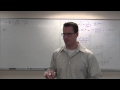Course

Calculus 2 by Leonard

4.75

(8)

Certificate

This course is free today and always will be, from start to finish you will pay nothing.Teacher

Leonard

This Channel is dedicated to quality mathematics education. It is absolutely FREE so Enjoy! Videos are organized in playlists and are course specific.

Course content preview

2h22m

0h44m

1h30m

1h15m

1h52m

1h16m

1h40m

1h54m

2h21m

2h09m

2h55m

2h48m

2h49m

2h27m

2h01m

1h11m

1h34m

1h19m

1h46m

Calculus 2 Lecture 9.7: Power Series, Calculus of Power Series, Ratio Test for Int. of Convergence

2h29mWatch the 1st video of the course

Do not worry, it is free!

Course content2h22m

Calculus 2 Lecture 6.1: The Natural Log Function

Calculus 2 Lecture 6.1: The Natural Log Function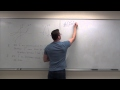0h44m

Calculus 2 Lecture 6.2: Derivatives of Inverse Functions

Calculus 2 Lecture 6.2: Derivatives of Inverse Functions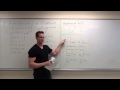1h30m

Calculus 2 Lecture 6.3: Derivatives and Integrals of Exponential Functions

Calculus 2 Lecture 6.3: Derivatives and Integrals of Exponential Functions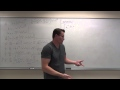1h15m

Calculus 2 Lecture 6.4: Derivatives and Integrals of General Exponential Functions

Calculus 2 Lecture 6.4: Derivatives and Integrals of General Exponential Functions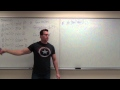1h52m

Calculus 2 Lecture 6.5: Calculus of Inverse Trigonometric Functions

Calculus 2 Lecture 6.5: Calculus of Inverse Trigonometric Functions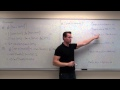1h16m

Calculus 2 Lecture 6.6: A Discussion of Hyperbolic Functions

Calculus 2 Lecture 6.6: A Discussion of Hyperbolic Functions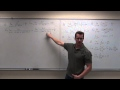1h40m

Calculus 2 Lecture 6.7: Evaluating Limits of Indeterminate Forms

Calculus 2 Lecture 6.7: Evaluating Limits of Indeterminate Forms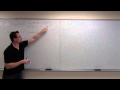1h54m

Calculus 2 Lecture 7.1: Integration By Parts

Calculus 2 Lecture 7.1: Integration By Parts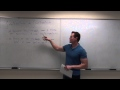2h21m

Calculus 2 Lecture 7.2: Techniques For Trigonometric Integrals

Calculus 2 Lecture 7.2: Techniques For Trigonometric Integrals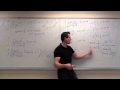2h09m

Calculus 2 Lecture 7.3: Integrals By Trigonometric Substitution

Calculus 2 Lecture 7.3: Integrals By Trigonometric Substitution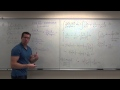2h55m

Calculus 2 Lecture 7.4: Integration By Partial Fractions

Calculus 2 Lecture 7.4: Integration By Partial Fractions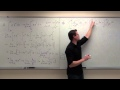2h48m

Calculus 2 Lecture 7.6: Improper Integrals

Calculus 2 Lecture 7.6: Improper Integrals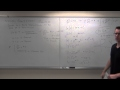2h49m

Calculus 2 Lecture 8.1: Solving First Order Differential Equations By Separation of Variables

Calculus 2 Lecture 8.1: Solving First Order Differential Equations By Separation of Variables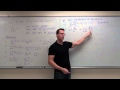2h27m

Calculus 2 Lecture 9.1: Convergence and Divergence of Sequences

Calculus 2 Lecture 9.1: Convergence and Divergence of Sequences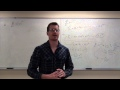2h01m

Calculus 2 Lecture 9.2: Series, Geometric Series, Harmonic Series, and Divergence Test

Calculus 2 Lecture 9.2: Introduction to Series, Geometric Series, Harmonic Series, and the Divergence Test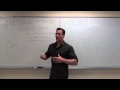1h11m

Calculus 2 Lecture 9.3: Using the Integral Test for Convergence/Divergence of Series, P-Series

Calculus 2 Lecture 9.3: Using the Integral Test for Convergence/Divergence of Series, P-Series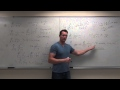1h34m

Calculus 2 Lecture 9.4: The Comparison Test for Series and The Limit Comparison Test

Calculus 2 Lecture 9.4: The Comparison Test for Series and The Limit Comparison Test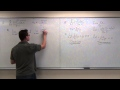1h19m

Calculus 2 Lecture 9.5: Showing Convergence With the Alternating Series Test, Finding Error of Sums

Calculus 2 Lecture 9.5: Showing Convergence With the Alternating Series Test, Finding Error of Sums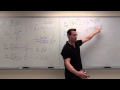1h46m

Calculus 2 Lecture 9.6: Absolute Convergence, Ratio Test and Root Test For Series

Calculus 2 Lecture 9.6: Absolute Convergence, Ratio Test and Root Test For Series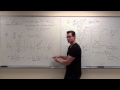2h29m

Calculus 2 Lecture 9.7: Power Series, Calculus of Power Series, Ratio Test for Int. of Convergence

Calculus 2 Lecture 9.7: Power Series, Calculus of Power Series, Using Ratio Test to Find Interval of Convergence